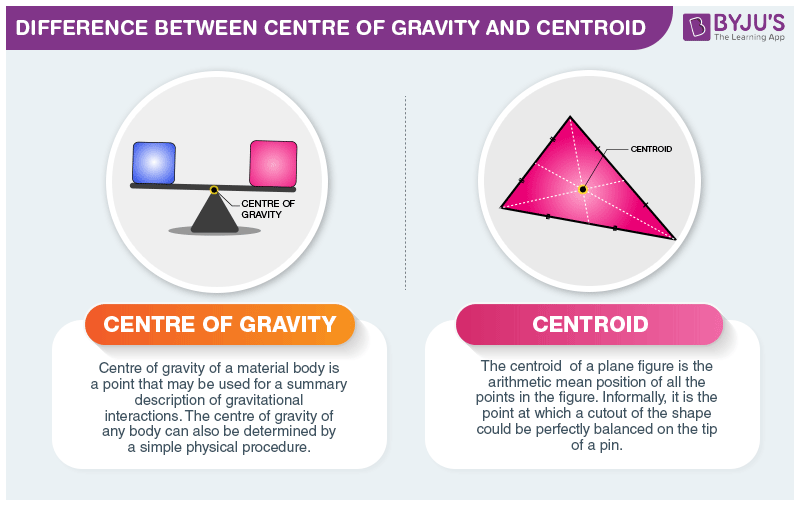# Difference Between Centre of Gravity and Centroid

Every object has some mass, and the object weighs a certain amount due to gravitational force. There is a difference between the centre of gravity and centroid. Both depend on the factor of uniform or non-uniform distribution of mass across the body.## Difference between Centre of Gravity and Centroid

In an object, a centre of mass is referred to as the point where the whole object’s mass is focused, which means the point’s mass is represented as the whole object’s mass. The centre of gravity of any object is the point where gravity acts on the body.

On the other hand, the centroid is referred to as the geometrical centre of a uniform density object. This means the object has its weight distributed equally across all body parts. If the body is homogeneous (having constant density), its centre of gravity is equivalent to the centroid.

Difference Between Centre of Gravity and Centroid
Centre of Gravity Centroid
The point where the total weight of the body focuses upon It is referred to as the geometrical centre of a body
It is the point where the gravitational force (weight) acts on the body It is referred to the centre of gravity of uniform density objects
It is denoted by g It is denoted by c
Centre of Gravity in a uniform gravitational field is the average of all points, weighted by local density or specific weight The centroid is a point in a plane area in such a way that the moment of area about any axis throughout that point is 0
It is a physical behaviour of the object, a point where all the weight of an object is acting It is a geometrical behaviour. It is the centre of measure of the amount of geometry.

These were some differences between centre of gravity and centroid. If you wish to find out more, download BYJU’S The Learning App.

RELATED ARTICLES:## Frequently Asked Questions – FAQs

Q1

### Define centre of gravity.

The point where the total weight of the body focuses upon is known as the centre of gravity. It is the point where the gravitational force (weight) acts on the body, it is denoted by g.
Q2

### Define centroid.

A centroid is the geometrical centre of a body. It refers to the centre of gravity of uniform density objects, denoted by c.
Q3

### What is the centre of mass?

Centre of mass of a body or system of a particle is defined as, a point at which the whole of the mass of the body or all the masses of a system of particle appeared to be concentrated.
Q4

### What is gravitational force?

Each body in this universe attracts other bodies towards itself with a force known as gravitational force, thus gravitation is a study of the interaction between two masses.
Q5

### Why are space rockets launched eastwards?

Earth spins (rotates) from west to east in 24 hours. If the space rockets are launched in the same direction, the relative velocity of the rocket increases which helps it to rise without much fuel.
Test your knowledge on Centre of gravity and centroid difference